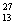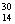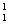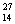Visitors Online: 77 | Friday 24th May 2019
 CBSE Guess > Papers > Question Papers > Class X > 2006 > Science > Delhi Set - I SCIENCE AND TECHNOLOGY— 2006 (Set I — Delhi)
SECTION - A Q. 1. What is the purpose of quenching steel?(1) Q. 2. What constitutes the field of a magnet? (1) Q. 3. What is the advantage of the third wire of earth connection in domestic electric appliances? (1) Q. 4. Name the process of large energy production in the Sun. (1) Q. 5. What is efflorescence? Give an example. (2) Q. 6. Draw a ray diagram to show the position and nature of the image formed when an object is placed between focus F and pole P of a concave mirror. (2) Q. 7. Give one example of each and state the role of a moderator and a coolant in a nuclear reactor. (2) Or Complete the following nuclear reaction equations:AI +He -------->Si + ………..AI +H --------->Si + ……....... Q. 8. If you observe the position of a star at a fixed time everyday, how many degrees higher will it appear after a month of 30 days? Describe an activity to show that a star moves a certain angle in the sky relative to its position the previous day. (2) Q. 9. An aqueous solution has a pH value of 7.0. Is this solution acidic, basic or neutral? If H+ concentration of a solution is 1 x 10-2 mol L-1 what is the pH value for it? Which has a higher pH value, 1 M HC1 or 1 M NaOH solution? (3) Q. 10. Describe with a labelled diagram, the Froth Floatation Process used to separate the gangue from a Sulphide ore. (3) Or Name a major ore from which iron is extracted. Write chemical equations for the reactions that take place in the blast furnace for the extraction of iron from this ore. Q. 11. Give reasons for the following: (3) Metals are regarded as electro-positive elements. When a piece of Copper metal is added to a solution of Zinc sulphate, no change takes place, but the blue colour of Copper sulphate fades away when a piece of zinc is placed in its solution. Articles made of Aluminium do not corrode even though aluminium is an active metal. (3) Q. 12. What is the ‘fermentation process’? Name the gas evolved during the fermentation process. List the two products formed when the enzyme invertase acts on sugar present in molasses. (3) Or Complete the following equations and write the names of products formed CH3COONa + NaOH -----> Heat CH3COCH3 + HCN --------------> CH3OH + O2 --------->Heat ag catalyst Q. 13. A 5.0 cm tall object is placed per pendicular to the principal axis of a convex lens of focal length 20 cm. The distance of the object from the lens is 30 cm. By calculation determine (i) the position, and (ii) the size of the image formed. (3) Q. 14. Draw a schematic diagram of a circuit consisting of a battery of five 2 V cells, a 5 ohm resistor, a 10 ohm resistor and a 15 ohm resistor, and a plug key, all connected in series. Calculate the electric current passing through the above circuit when the key is closed. (3) Q. 15. Why is Sulphuric acid called the ‘king of chemicals’? Name the gas evolved when: Concentrated Sulphuric acid acts on Sulphur. Dilute Sulphuric acid acts on Sodium carbonate. State the colour change you would observe on adding concentrated Sulphuric acid to: Blue Copper sulphate crystals Colourless cane-sugar crystals. (5) Q. 16. Distinguish between renewable and non-renewable sources of energy giving one example of each. Why is the use of wood as a fuel not advised although forests can be replenished? Or Write the value of solar constant of Sun. What type of mirror is used in Box Type Solar Cooker? Why it is difficult to use hydorgen as a source of energy? What is the maximum temperature attained in a concave reflector type solar cooker? Fossil fuels are classified as non renewable source of energy. Explain why. SECTION - B Q. 17. What is breathing? (1) Q. 18. Name the type of blood vessels which carry blood from organs to the heart. (1) Q. 19. What is meant by a biodegradable waste? Which of the following is/are biodegradable? (2)        Agricultural residue, Plastics, Insecticides, Ssewage. Q. 20. Suggest any two ways to strike a balance between environment and development. (2) Q. 21. Draw the diagram of a palisade cell of a plant leaf and label the following in it (3) Chioroplast Vacuole Cytoplasm Nucleus. Q. 22. Describe the central nervous system in human beings under the following heads: Regions included Three functions of any one region. (3) Q. 23. When is blood clotting useful? In a flow chart illustrate the four major events involved in blood clotting. (3) Q. 24. What is ‘genetics’? (5) Give the common name of the plant on which Mendel performed his experiments. What for did Mendel use the term factors and what are these factors called now? What are genes? Where are the genes located? Or Who provided the evidence of DNA as a genetic material? Why is DNA called as polynucleotide? List the three important features of double helical model of DNA. Science 2006 Question Papers Class X Delhi Outside Delhi Compartment Delhi Compartment Outside Delhi Set I Set I Set I Set I Set II CBSE 2006 Question Papers Class X